# 第2课第6节_Java面向对象编程_包和权限_P【学习笔记】

1、使用package定义编译的时候存放的位置

```1 package a.b.c.d;
2
3 public class Package {
4     public static void main(String args[]){
5         System.out.println("Hello,World");
6     }
7 }```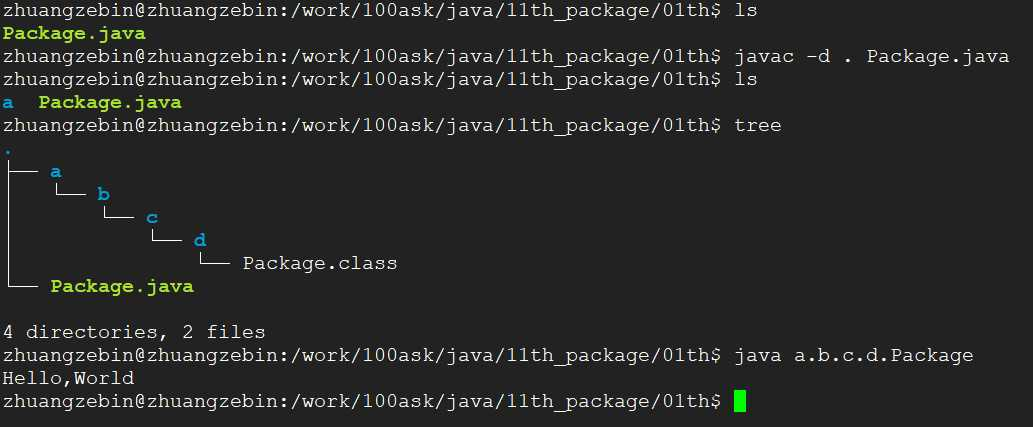2、下图大致写出了引入包的作用，假如有两个人写了同样的一个类Math类，里面实现了不同的运算方法，我们可以通过目录来区分开来。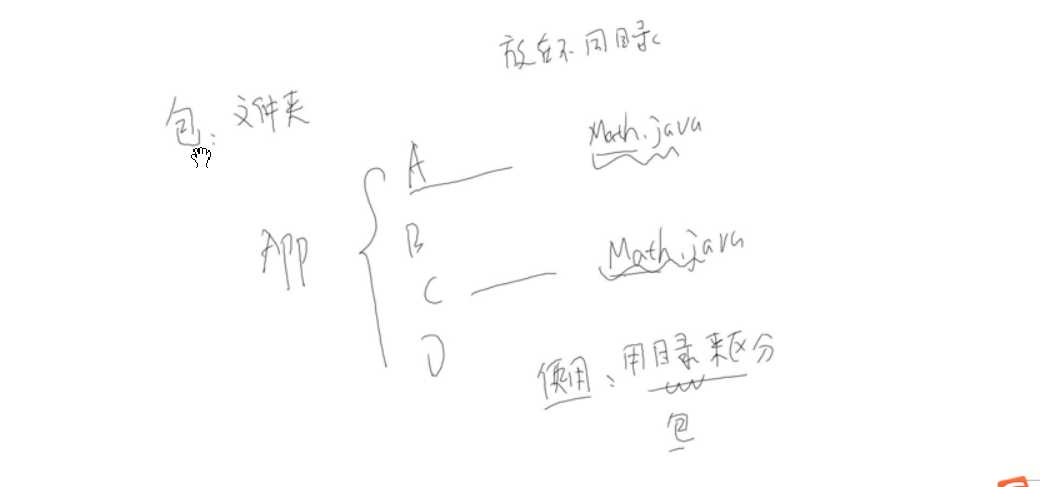lisi的Math.java代码如下

```1 package a.b.c.d1;
2
3
4 public class Math{
5     public static int add(int x,int y){
6         return x + y;
7     }
8 }```

zhangsan的Math.java代码如下:

```package a.b.c.d2;

public class Math{
public static int add(int x,int y){
return x + y + 2;
}

public static int sub(int x,int y){
return x - y;
}
}```

Package.java代码如下

``` 1 import a.b.c.d1.*;
2 import a.b.c.d2.*;
3
4 public class Package {
5     public static void main(String args[]){
8
9         System.out.println(a.b.c.d2.Math.sub(1,2));
10     }
11 }```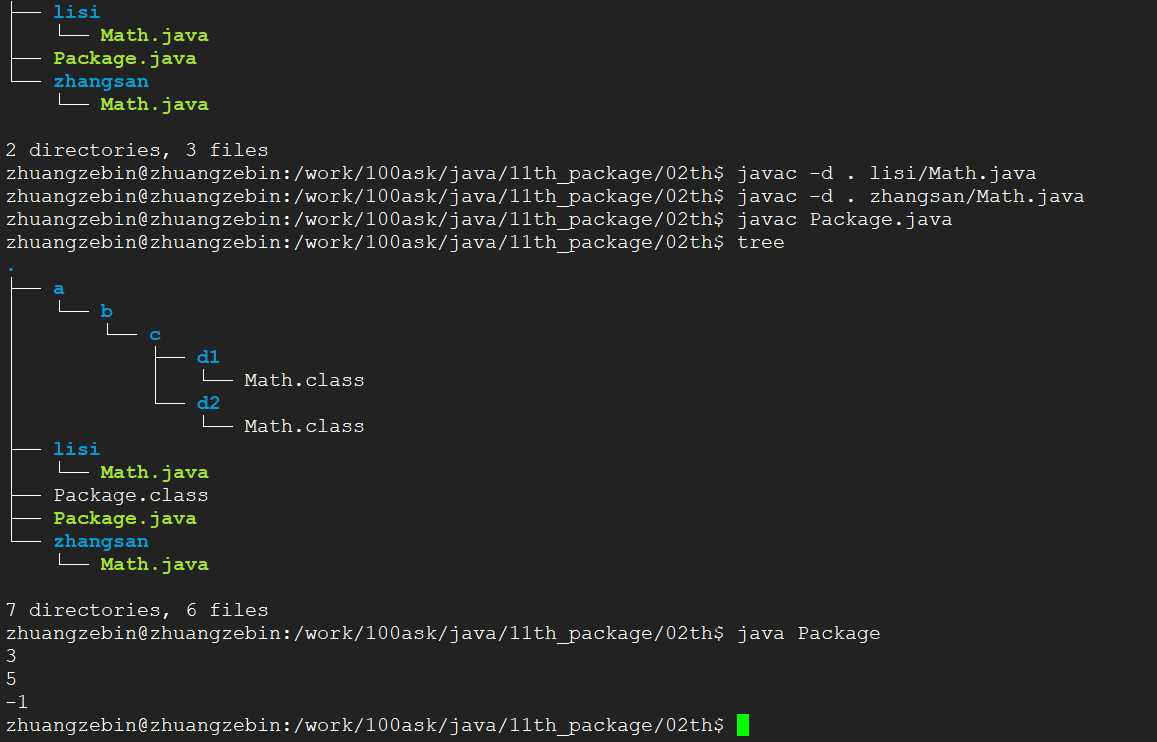3、在上述的代码基础上在张三目录添加Print.java类，用于打印信息，在Package.java类中，可以通过包名来使用类的方法，如果该方法没有同名的类方法，可以不使用包名直接使用该方法.

Print.java

```1 package a.b.c.d2;
2
3
4 public class Print{
5     public static void printInfo(){
6         System.out.println("package a.b.c.d2;");
7     }
8 }```

Package.java

``` 1 import a.b.c.d1.*;
2 import a.b.c.d2.*;
3
4 public class Package {
5     public static void main(String args[]){
8
9         System.out.println(a.b.c.d2.Math.sub(1,2));
10
11         a.b.c.d2.Print.printInfo();
12         Print.printInfo();
13     }
14 }```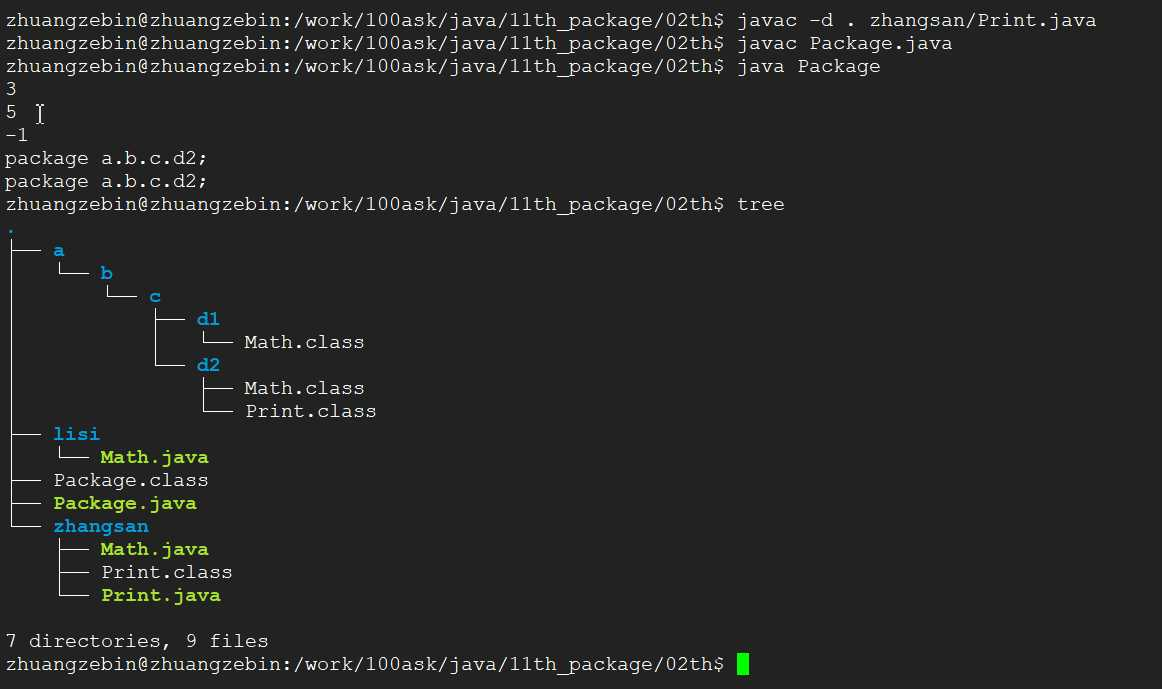4、张三、李四开发的代码，在编译之后都生成在a目录下面，我们可以把a目录打包成jar包之后，给用户，这样也可以不用开源自己的代码。使用jar把a目录压缩成jar包，并且在环境变量中添加my.jar包，主程序Package就可以使用，张三和李四实现的方法了。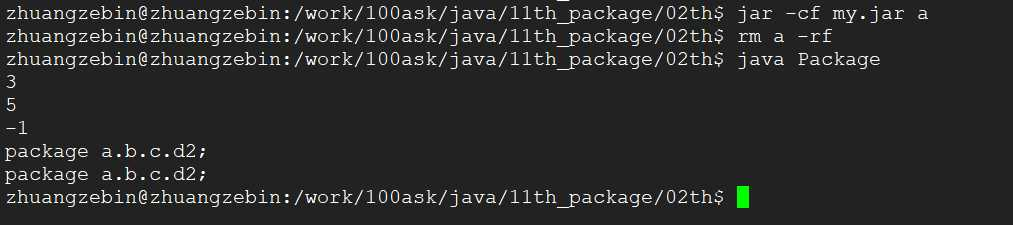5、访问权限,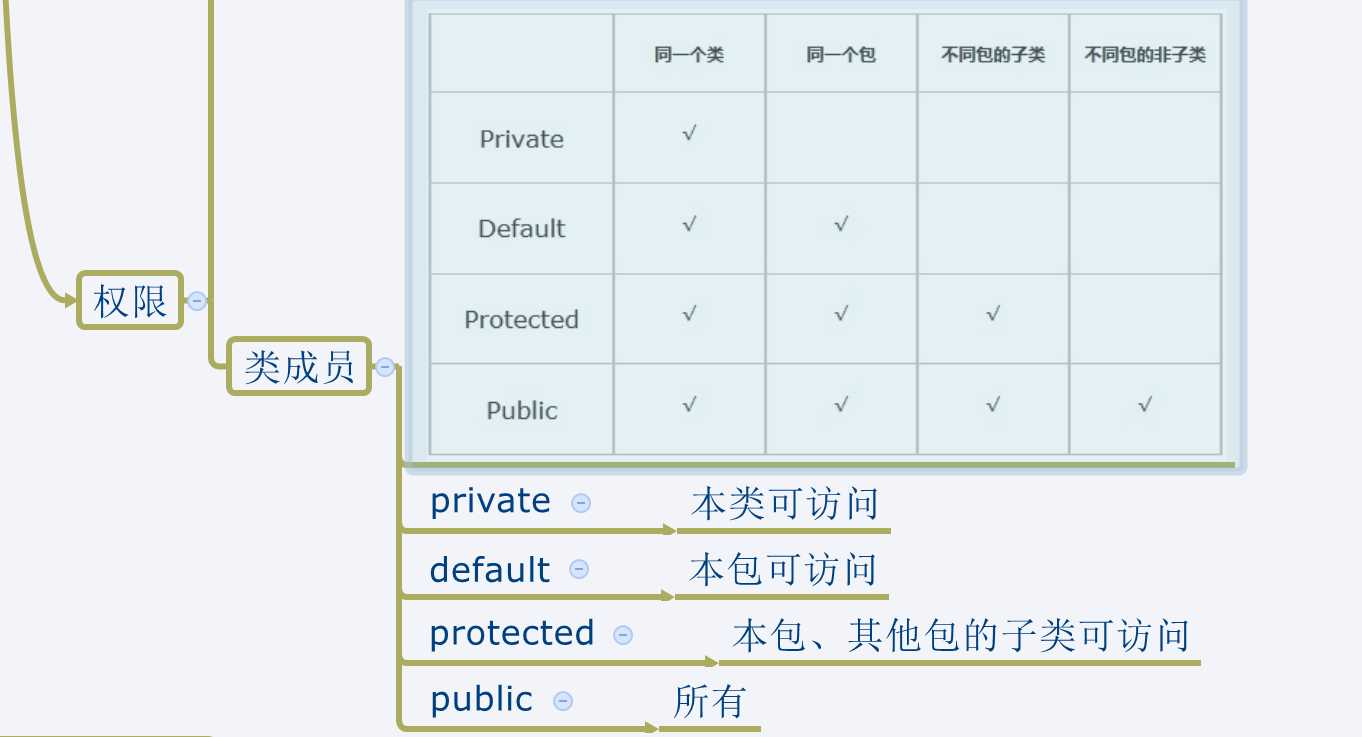public class可以被外包访问,class只能在本包中访问

Mymath.java

```1 package b;
2
3 //class Mymath{  编译失败
4 public class Mymath{
5 }```

Pack.java

```1 package a;
2
3 public class Pack{
4     public static void main(String args[]){
5         Mymath m = new Mymath();
6     }
7 }```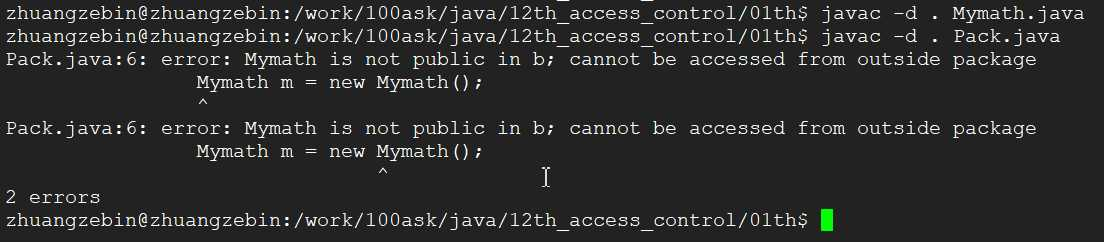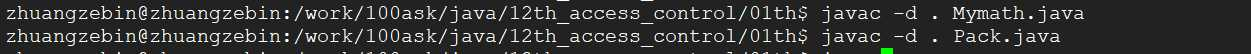6、将5的代码Mymath类也定义在包a中，那样Mymath没有加public也可以在pack类中访问

Mymath.java

```1 package a;
2
3 class Mymath{
4
5 //public class Mymath{
6
7 }```

Pack.java

```1 package a;
2 //import b.*;
3
4 public class Pack{
5     public static void main(String args[]){
6         Mymath m = new Mymath();
7     }
8 }```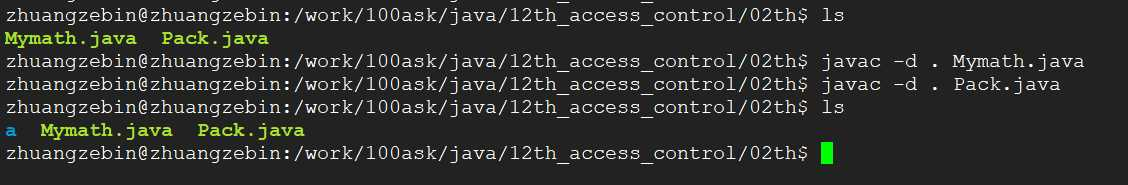7、同一个包中，一个类的属性定义为private，无法被另外一个类访问。去掉private为默认属性，可以被另外一个类访问。

Mymath.java

```1 package a;
2
3 class Mymath{
4     private int x;      //int x;     去掉private则可以编译通过
5 }```

Pack.java

```1 package a;
2 //import b.*;
3
4 public class Pack{
5     public static void main(String args[]){
6         Mymath m = new Mymath();
7         m.x = 0;
8     }
9 }```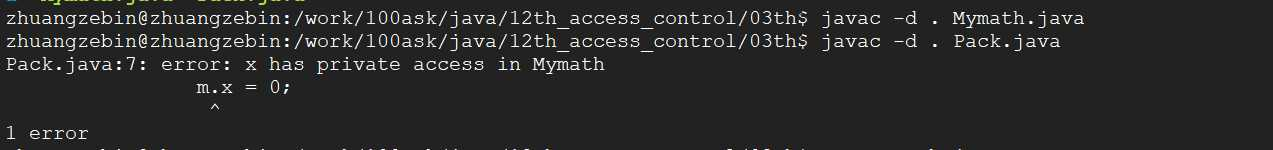8、使用protect定义属性，本包、其他包的子类可访问

Mymath.java

```1 package b;
2
3 public class Mymath{
4     protected int x;
5 }```

Pack.java

``` 1 package a;
2 import b.*;
3
4 class Mysubmath extends Mymath{
5     void printInfo(){
6         System.out.println("Mysubmath: x = " + x);
7     }
8 }
9
10 public class Pack{
11     public static void main(String args[]){
12         Mysubmath m = new Mysubmath();
13         //m.x = 0;
14         m.printInfo();
15     }
16 }```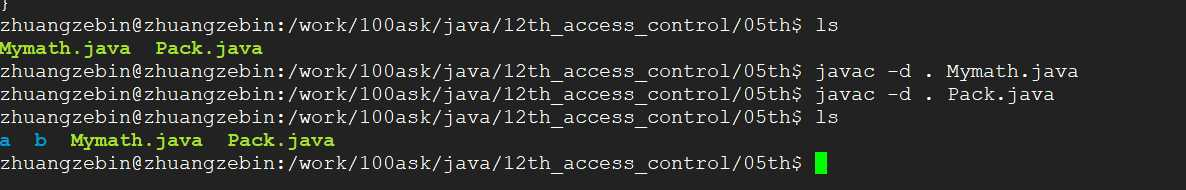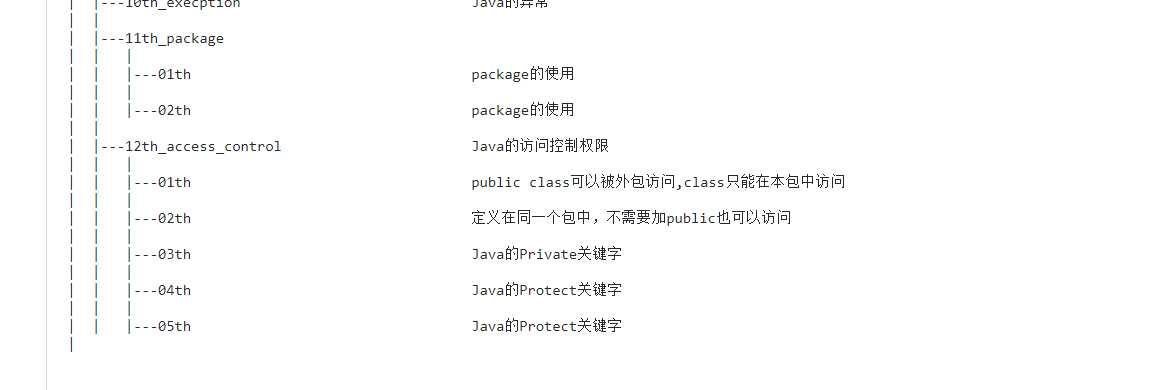(0)
(0)

© 2014 bubuko.com 版权所有 鲁ICP备09046678号-4